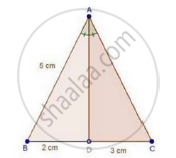Share

In a δAbc, Ad is the Bisector of ∠A, Meeting Side Bc at D. If Bd = 2cm, Ab = 5cm and Dc = 3cm, Find Ac. - CBSE Class 10 - Mathematics

Question

In a ΔABC, AD is the bisector of ∠A, meeting side BC at D.

If BD = 2cm, AB = 5cm and DC = 3cm, find AC.

SolutionWe have,

AD is the bisector of ∠A

We know that, the internal bisector of an angle of a triangle divides the opposite side internally in the ratio of the sides containing the angle.

therefore"BD"/"DC"="AB"/"AC"

rArr2/3=5/"AC"

rArr"AC"=(5xx3)/2=15/2

⇒ AC = 7.5 cm

Is there an error in this question or solution?

Video TutorialsVIEW ALL 

Solution In a δAbc, Ad is the Bisector of ∠A, Meeting Side Bc at D. If Bd = 2cm, Ab = 5cm and Dc = 3cm, Find Ac. Concept: Angle Bisector.
S